## sábado, 2 de mayo de 2015

### Charging and discharging of a capacitor

To see this phenomenon we need a data acquisition device, in this case we have taken the National Instruments NI / USB device 6008. This phenomenon leads us as a signal, as all analog signals are level, shape and frequency. The signal shapes are sine, square, triangle. After trasnductor have to condition the signal and then it acquires. Many transducers are integrated conditioner. But it takes one. The external amplifier must be attached to the low level signal, if separated increase errors. Within the DAQ there is a new amplifier that is internal.
To prevent noise, short cables, shielded and twisted. Non-rigid and flexible and are welded better for signal acquisition, in our there is a 10% error level is tolerable.
The SNR is the relationship that I have between the signal to noise enters and is better than most that the internal amplified external amplifier to make the relationship as fair to the measure.
Not good solder wires creates precision impedance and if we have to grapinar or crimp but the data that we are wrong. Resolution of an ADC is expressed in number of bits and when ranking is more expensive.
The amplification depends on the range to be set, ie if the signal varies from 0-2 volts have therefore adjusted the DAQ 0-2 volts.
We must differentiate to connect the DAQ differentially or RSE. If the device is CSR reference and if the NRSE source.
If you connect to the computer USB reference to the DAQ ours is RSE, as long as the computer is connected by cord, if you remove the battery cable and throw things change. The chassis are all grounded and devices to GND.
You have to look at the ground reference for the DAQ not fuck because land lines are not equal. The problem of land and no potential difference is that puts RSE is not recommended. To avoid this the device remove the device ground reference. The RSE is used to signal more than 1 volt and short cables.
NRSE long cable is used and less than one volt, so is difference it is with two potential. If the signal is floating need a return pull down resistors are used to measure floating signals externally as a thermocouple.
We must explain that is the Aliasing is we want to measure a signal with a higher frequency that I purchase. Nyquist has simple formula to know if we aliasing and how often you would get in the case. To avoid this ensures that the measurement range should not exceed half the total that can DAQ, with east to 5 kHz and yours would be a 1kHz.
DAQ RAM is 512 bytes FIFO has an input of + - simple and + 10v -20 differential mode so you can put +10 and -10 and an impedance of 147K, which is for user level, although for more serious things would not be.
It has an external trigger, differential mode more accurately. It has two analog outputs and a resolution of 12 bits but we'll use 11 bits to give customers security as it varies. It is updated every 150Hz. which can generate signals about 6 milliseconds. Analog output of 0-5 volts and impedance of 50ohms.
5mA current, requires an interface or operating a follower for peta but should not go alone. Slew rate, the rate of change in a microsecond, can at most only 1 microsecond one volt, supports a short 50mA. Digitalemente two ports August 1 lines and one of 4. It is open collector or drain, we need a 10k pull up to take signals. Taking to 0 from -0.3 to 0,8 logic 0 voltage having a 32-bit counter.
Maximum pulse width of 100ns both upstream as downstream.
After entering the data from our DAQ to roughly the device image detail.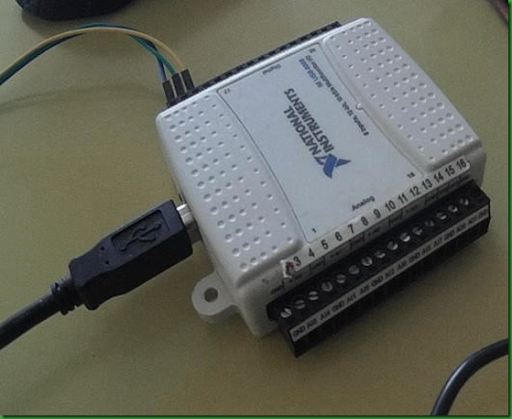Apart from this device need a device that will help us take these signals in what will our capacitor, resistor loading and unloading, to have done it with the Proteus software design. This design will make the connection so RSE exceed one volt but have less accuracy.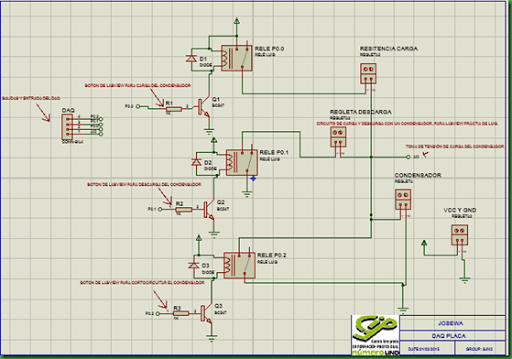If you want to do differential.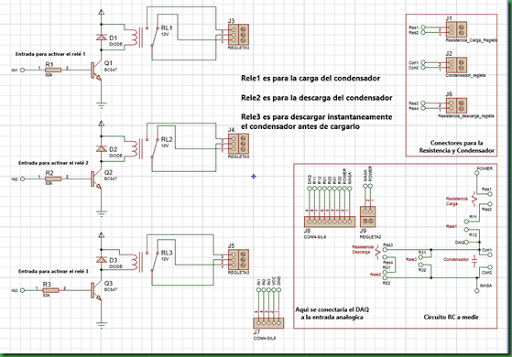This is the board that will in the CNC. We need, three relays,, four strips, headers, 8K2 three resistors, three transistors and three diodes 1N4001 BC547.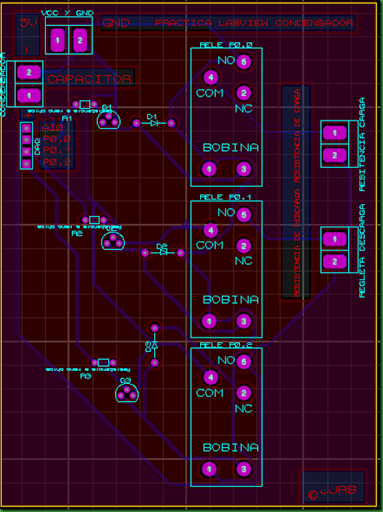And differential.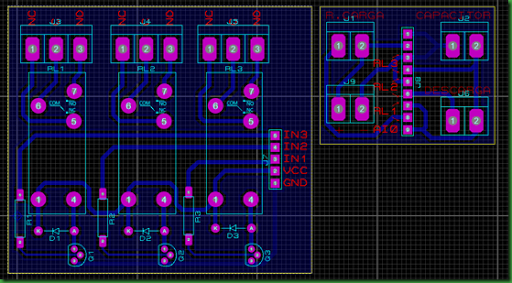We carry the plate and weld the components being in this way.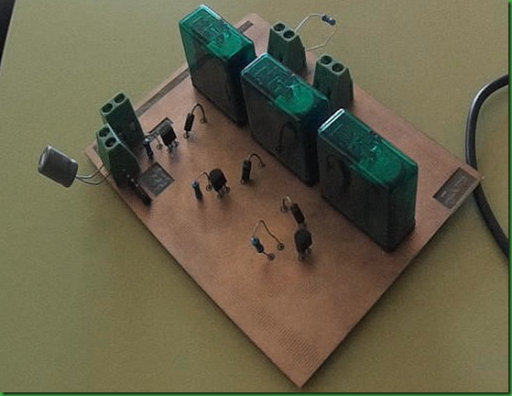And differential.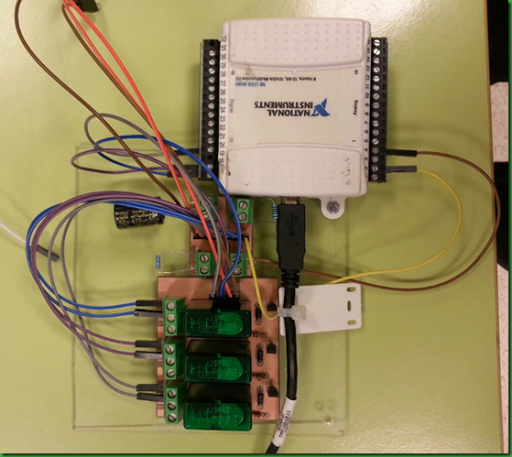Proceed to connections, this is RSE, although if we connect to a desktop computer is not recommended because it would be in the same earth, you better take Differential in our case as we will go with the laptop without connecting to food only with batteries, there is no problem.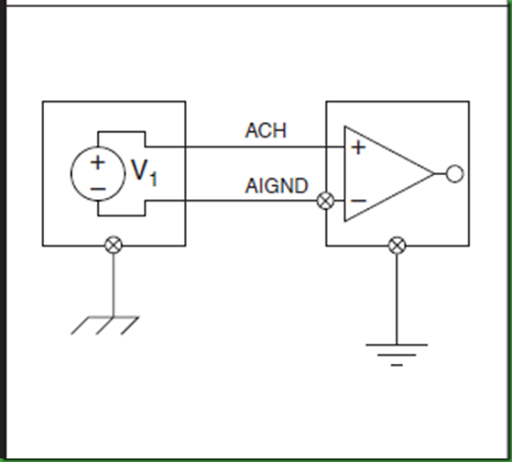If we do it with desktop pc resort to differential. which is far better method as attached image.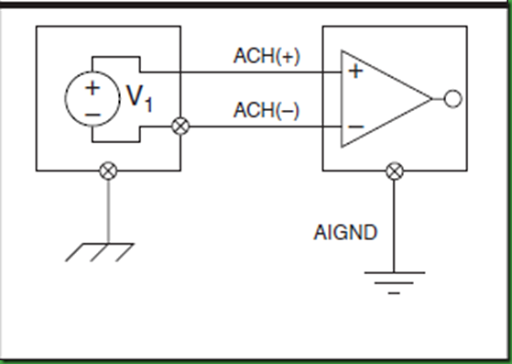We need first of all to calculate the value of the final charge on the capacitor, how long it takes to charge the capacitor 99.6% of the final charge, so we must use the formula that will be using constantly Labview to calculate the ideal capacitor for our practice. As shown we will teach how to calculate the charging time of the capacitor in 5tau which is what is considered to be almost fully charged. First a brief outline of the capacitor charge and then calculations.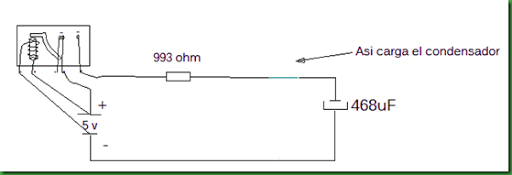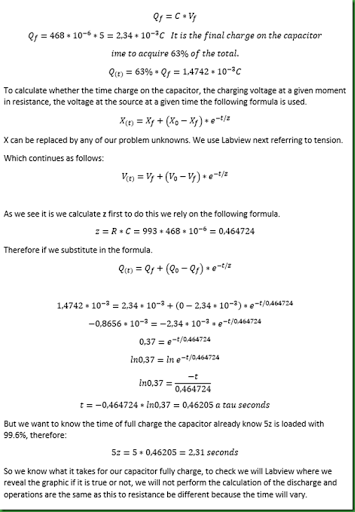We first present our interface and then we'll explain the code.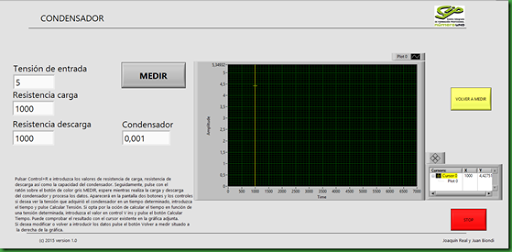In input voltage will stress that has the power, you must use two power supplies, one for the condenser and one for the relay in this way do not have a voltage drop causing an inaccuracy in data entry (This differential mode), to find the right entry have used a voltage controlled voltage source for both excitation of the relays and to the capacitor. As we have used RSE have this problem but hardly touching but gives an inaccuracy 10 millivolts.
We started with the code. Add that if we have a DAQ connected to your computer via USB we can not do the practice we also have to be installed in the package Measurement I / O-NI DAQmx
We put it in a while an event which starts when you press the "MEASURE" button then will be explained more closely.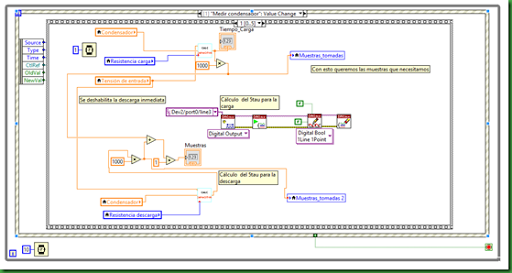The following is if the Stop button is pressed, makes a stop on our VI.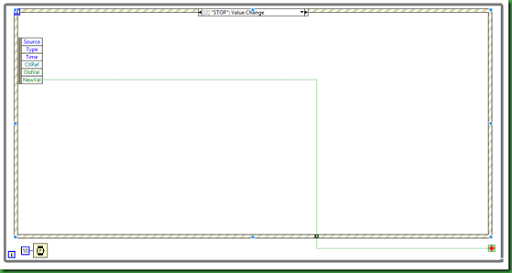If we give "Volver a medir" empty values stored in the graphic and leave everything so that we can re-enter data.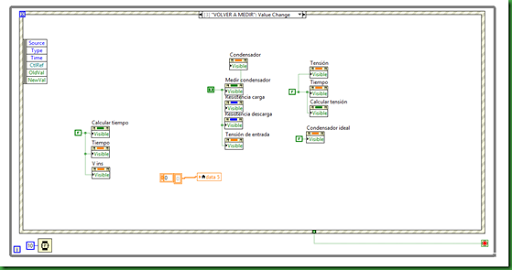The next step is to calculate voltage, find a point on the graph versus time.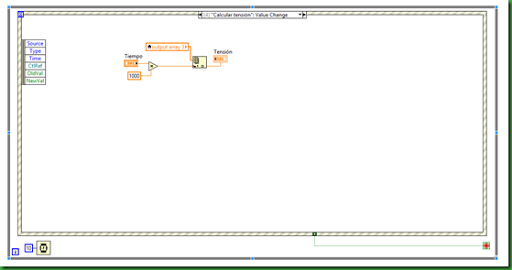The latter function calculates the instantaneous voltage of the capacitor charging time.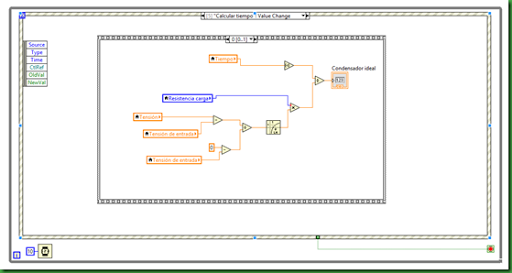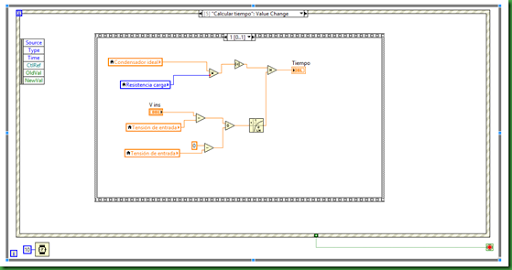Once generally seen the code, we will not enter to see how we measure the DAQ.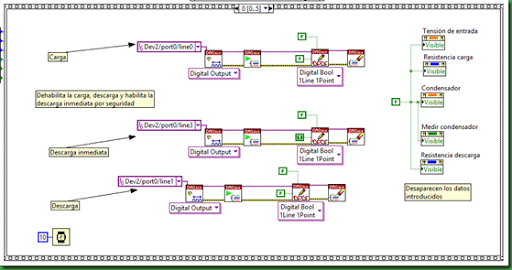Once you run this frame moves to the next that disables the immediate download and performs calculations to know the capacitor charge in 5tau to determine the number of samples to be taken by our DAQ, the more samples taken is better because they are points and sum of many points drawing perform charging capacitor. Similarly it is performed with the discharge resistor.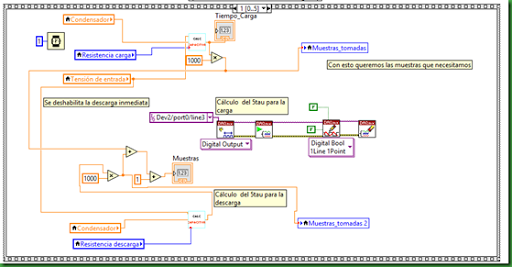Image as subVI performs calculations based on the formula that we have explained above enclosed.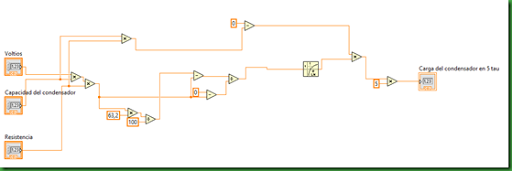The next frame determine that it is RSE, the AI0 input we want to take voltage samples I draw are 1000 start saying they are analog and created an array of DBL from one dimension to keep them at the same time I enable the load to go taking that data and store.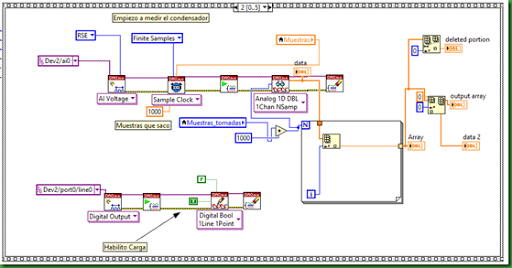And Differential.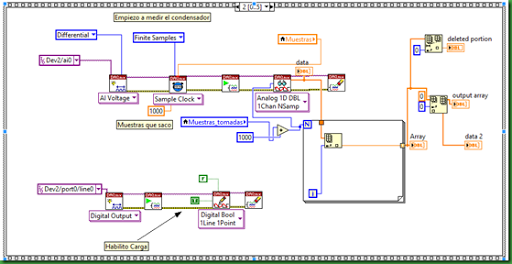Once I took the load data, the load becomes disabled in the following frame.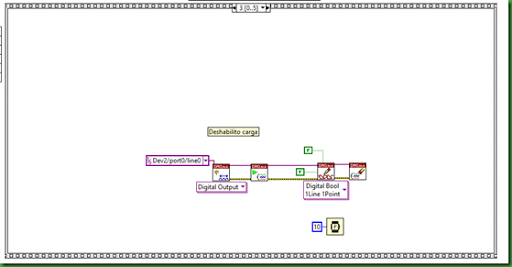In the next frame I enable downloading and at the charge and determine the number of samples and the data load and I keep.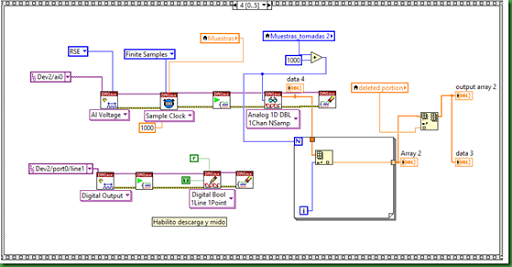And differential.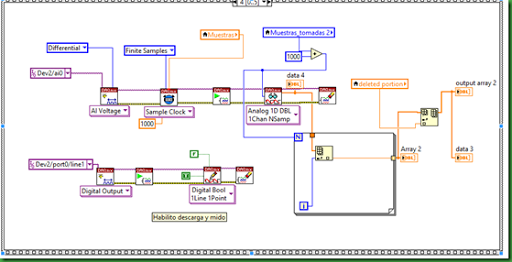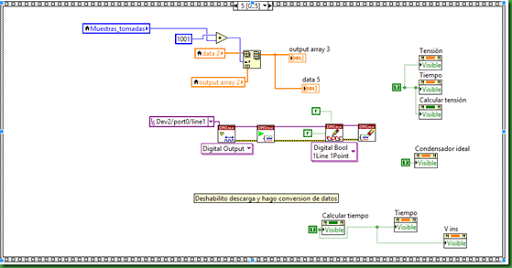This would be the end and leave you video of the practice, and I leave to you to see the results with the DAQ. The conclusion we can draw is that this system if it serves to know the details of the cargo but not used for the download because even pass some time between loading and unloading do not really know that charging is in charge if because we short-circuit in the capacitor. For these problems we've had to make other saw which calculate the ideal capacitor that you must put in the beginning of this saw, because if would gain real data will not match.
We explain the new I saw that you have attached, in him the ideal capacitor is calculated that if you put in the condenser of another saw you will fit in all the results, it put the value of the load resistor and capacitor value of Fluke and the final voltage. In time we put the charging time calculated by the formula. We give and we run the saw calculates the instantaneous voltage, put that value into the hollow of V ins and give us a run and takes the time as Vins, put the time in Time for calculation condenser and back to give run and calculates the capacitor we must put in the main we saw it we also estimated to have a value in 5tau clarification.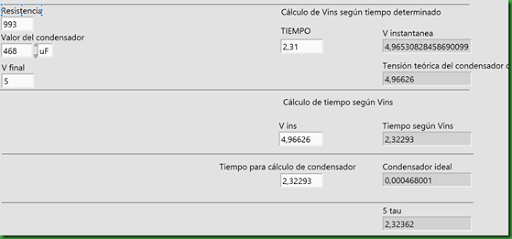To explain the code as we build on the formula that we have developed.
We chose a Flat sequence so that it could better understand. With this instantaneous voltage is calculated.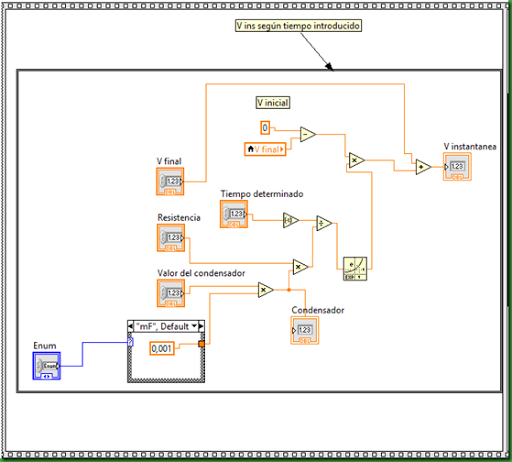Once the instantaneous voltage obtained calculate the time.Then the ideal time for a given capacitor.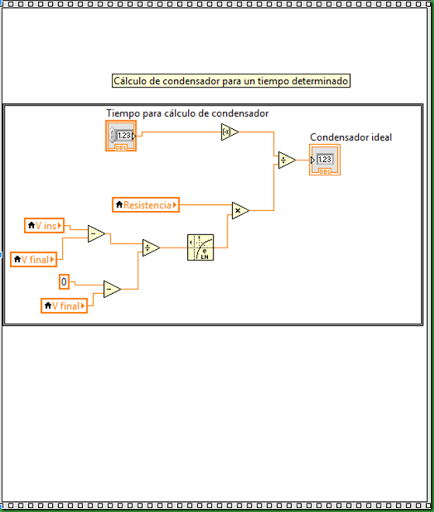And by the end the 5tau.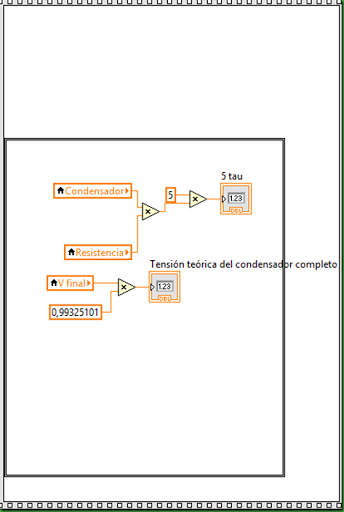If they give the following drawing may download all practice and see the graph of the capacitor.

#### 2 comentarios:

1.Wow, this is great.
Do you think you should use a quạt trần đèn?
quạt trần đèn trang trí loại nào tốt

2.Fabulous blog! Really each and every blog describe very clearly. Must visit at Nylon Sandwich Belts